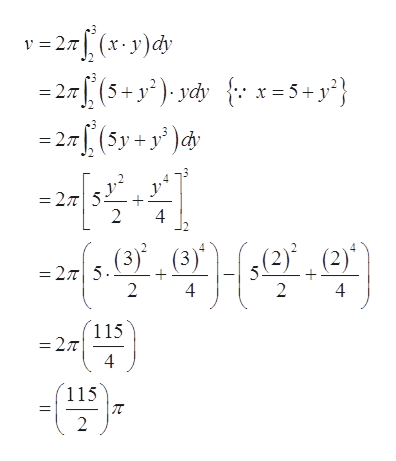# Use the method of cylindrical shells to find the volume V of the solid obtained by rotating the region bounded by the given curves about the x-axis.x = 5 + y2, x = 0, y = 2, y = 3

Question
38 views

Use the method of cylindrical shells to find the volume V of the solid obtained by rotating the region bounded by the given curves about the x-axis.

x = 5 + y2, x = 0, y = 2, y = 3

check_circle

Step 1

To determine the volume of solid by using the method of cylindrical shell.

Step 2

Curve equations are given as, x=5+y2, x=0, y=2, y=3 and about x-axis.

Step 3

Let the radius is y, height is x, then

The volume of solid by rotati...help_outlineImage Transcriptionclose27 (x-y)dy =27(5+y2) ydy = 27, (5y + y' )dv x=5+y*} = 27T 5 2 4 2) (2 =275. (3) (3) 2 4 2 115 =27 4 115 2 fullscreen

### Want to see the full answer?

See Solution

#### Want to see this answer and more?

Solutions are written by subject experts who are available 24/7. Questions are typically answered within 1 hour.*

See Solution
*Response times may vary by subject and question.
Tagged in

### Integration